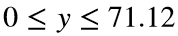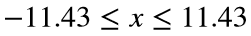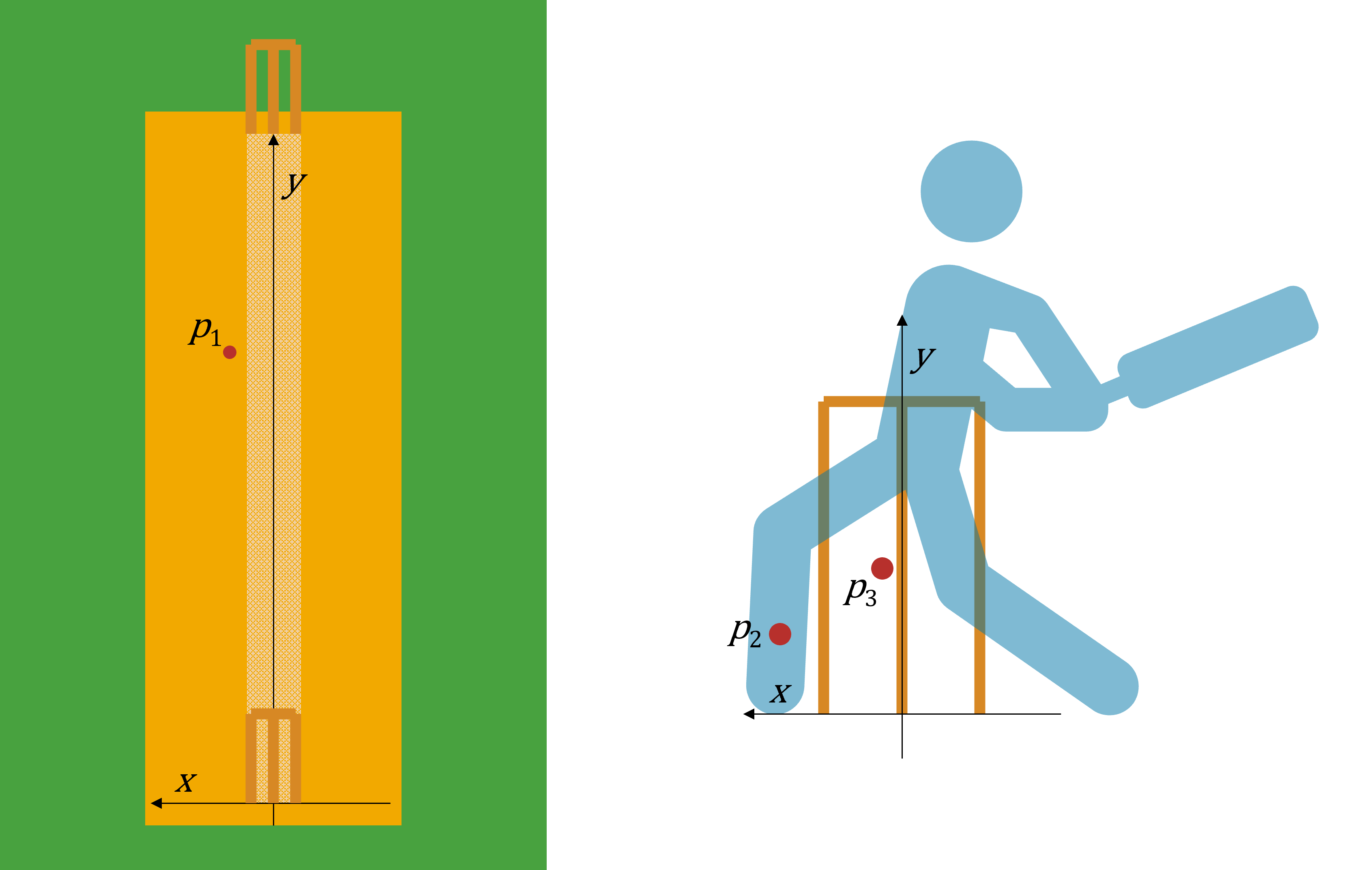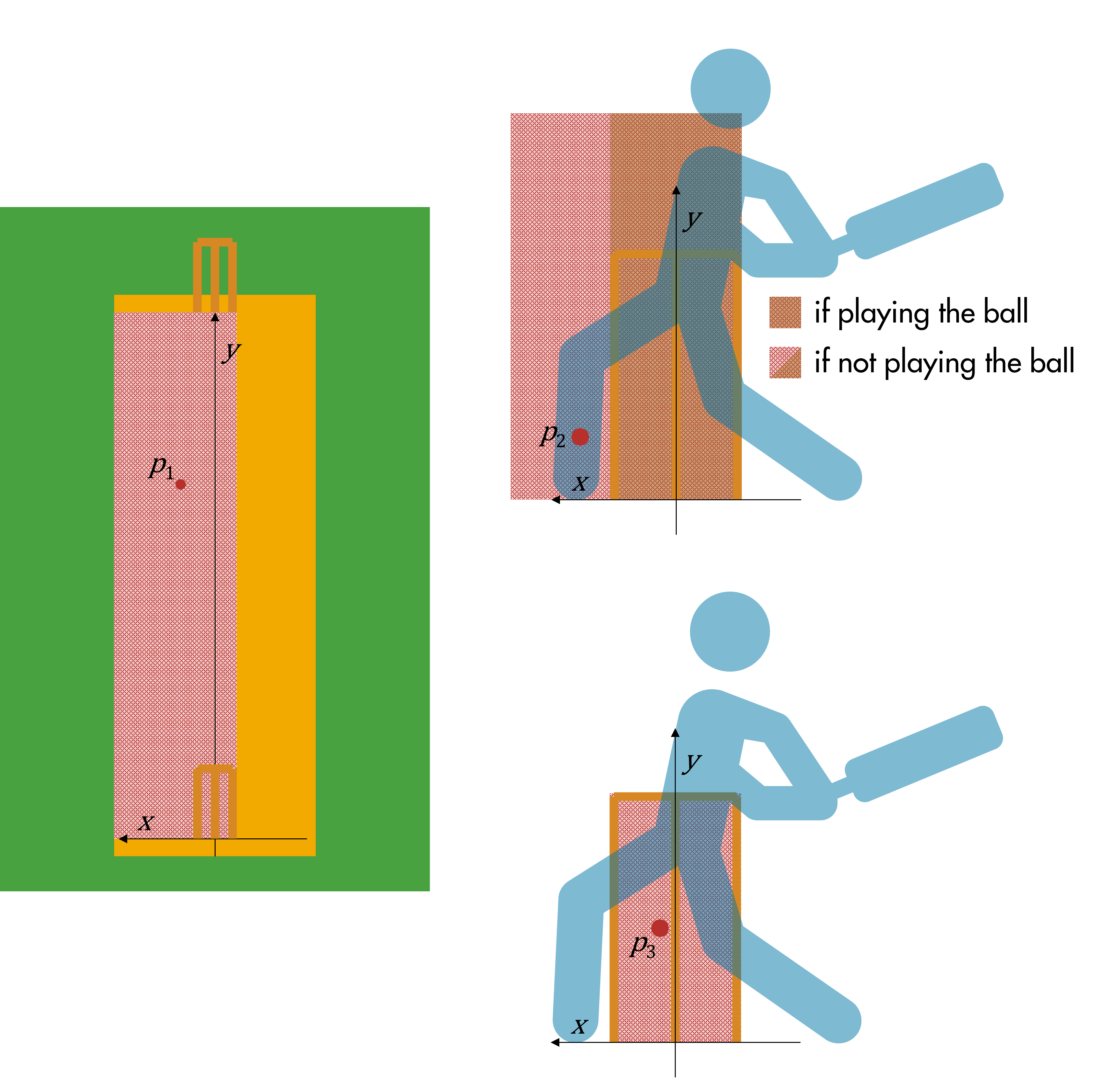# Problem 56543. Cricket - Is It LBW?

LBW has an unfair reputation as complicated. But if you've watched a third umpire referral, you'll know that it's just a logical process, based on four decisions: did the ball hit bat or pad first, where did the ball pitch, where was the impact with the pad, would it have hit the stumps? Given three (x,y) coordinate pairs representing the location of where the ball pitched, hit the batter, and would have hit the plane of the wicket, and a logical (T/F) scalar indicating whether the batter was attempting to play the ball, return a logical scalar indicating whether the batter is out LBW (assuming all the other criteria have been met, such as legal delivery, contact with the body before the bat, etc.)
Each coordinate pair is given as a 2-element vector [x y], where the x coordinate is the distance across the pitch, centered on middle stump, with x > 0 corresponding to the batter's off side (and x < 0 corresponding to the batter's leg side).
For the first coordinate pair - ie where the ball pitched - the y coordinate is measured from the bowler's wicket to the batter's wicket (hence 0 < y < 2012). If the ball did not pitch at all, this coordinate vector will be [NaN NaN].
For the other two coordinate pairs, the y coordinate is measured as height above the ground.
For simplicity, you can assume the wicket is a simple rectangle 71.12cm high (ie) and 22.86cm wide (ie).
All distances are in cm.(Diagram assumes a right-handed batter)
Law 36 (Leg Before Wicket) states that for the batter to be out LBW:
• The ball cannot pitch outside the line of leg stump
• If the batter is making a genuine attempt to play the ball, the impact has to be in line with the wicket (but can be above the height of the wicket)
• If the batter is not making an attempt to play the ball, the impact has to be in line with the wicket or outside the off stump
• The ball must have been going on to hit the wicket% pitching in line; impact in line (below stump height); playing the ball; hitting wickets => Out
p1 = [4.41 1385.29];
p2 = [-3.03 4.62];
p3 = [-6.3 9.71];
out = islbw(p1,p2,p3,true)
out =
logical
1

### Solution Stats

53.57% Correct | 46.43% Incorrect
Last Solution submitted on Nov 03, 2023